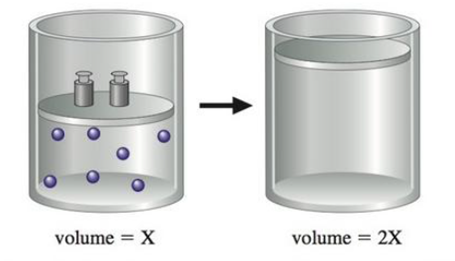# You have a gas in a container fitted with a piston, and you change one of the conditions of the gas such that a change takes place, as shown below: State three distinct changes you can make to accomplish this, and explain why each would work.### Chemistry: An Atoms First Approach

2nd Edition
Steven S. Zumdahl + 1 other
Publisher: Cengage Learning
ISBN: 9781305079243

#### Solutions

Chapter
Section### Chemistry: An Atoms First Approach

2nd Edition
Steven S. Zumdahl + 1 other
Publisher: Cengage Learning
ISBN: 9781305079243
Chapter 8, Problem 106E
Textbook Problem
4 views

## You have a gas in a container fitted with a piston, and you change one of the conditions of the gas such that a change takes place, as shown below:State three distinct changes you can make to accomplish this, and explain why each would work.

Interpretation Introduction

Interpretation: For the given picture, three distinct changes to double the volume of a container should be explained.

Concept introduction:

• A gaseous law with interpreting the relationship between the pressure and volume of a gas at constant temperature. This law is known as Boyle’s law.

Mathematically,

P1V

or

PV=K

Where,

P = pressure in atmospheres

V= volumes in liters

K= a constant for a particular gas at given temperature

• A gaseous law with interpreting the relationship between the temperature and volume of a gas at constant pressure. That is the behavior of gas towards the heat. This law is known as Charles’s law.

Mathematically this law can be written as,

VT

V=bT

Where,

T is temperature and b is proportionality constant

• A gaseous law with interpreting the relationship between the number of moles and the volume of a gas at constant temperature and pressure. This law is known as Avogadro’s law

Mathematically this law can be written as,

Vn

V=a n

Where,

n is the number of moles of gas and a is proportionality constant

### Explanation of Solution

Explanation

• To explain: the temperature change results in the larger volume.

According to Charles’s law at constant temperature, the volume of container is directly proportional to the temperature of gas.

This direct proportionality of temperature and volume can be can be explained as when temperature of a gas container is doubled, the kinetic energy of atomic gaseous molecules is increases and the gas molecule collide more frequently with walls of the container as a result the volume of container is doubled.

• To explain: the pressure change results in the larger volume.

According to Boyle’s law at constant temperature the volume of container is inversely proportional to the excreted pressure.

Volume of gas container is doubled by decrease in the external pressure at constant temperature. This direct proportionality can be explained as, when a gas kept in the particular container at constant temperature, the excreted pressure is inversely proportional to the volume of the container...

### Still sussing out bartleby?

Check out a sample textbook solution.

See a sample solution

#### The Solution to Your Study Problems

Bartleby provides explanations to thousands of textbook problems written by our experts, many with advanced degrees!

Get Started

Find more solutions based on key concepts
The dietary monosaccharides include. sucrose, glucose, and lactose fructose, glucose, and galactose galactose, ...

Nutrition: Concepts and Controversies - Standalone book (MindTap Course List)

25. Name each compound:

Chemistry In Focus

Explain why CH3NH2 is a Brnsted base.

Chemistry for Today: General, Organic, and Biochemistry

What is physically exchanged during crossing over?

Human Heredity: Principles and Issues (MindTap Course List)

What is a phosphoryl group?

General, Organic, and Biological Chemistry

A piece of copper is dropped into a beaker of water, (a) If the waters temperature rises, what happens to the t...

Physics for Scientists and Engineers, Technology Update (No access codes included)

Can the Sun ever become a black hole? Why or why not?

Foundations of Astronomy (MindTap Course List)